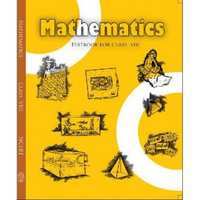Share

NCERT solutions for Class 8 Mathematics chapter 16 - Playing with Numbers

Mathematics Textbook for Class 8

NCERT Mathematics Class 8Chapter 16: Playing with Numbers

Ex. 16.10Ex. 16.20

Chapter 16: Playing with Numbers Exercise 16.10 solutions [Pages 255 - 256]

Ex. 16.10 | Q 1 | Page 255

Find the values of the letters in each of the following and give reasons for the steps involved.

3 A

+  2 5

-------

B 2

-------

Ex. 16.10 | Q 2 | Page 255

Find the values of the letters in the following and give reasons for the steps involved.

4 A

+ 9 8

------

C  B 3

------

Ex. 16.10 | Q 3 | Page 255

Find the value of the letter in the following and give reasons for the steps involved.

1 A

x A

------

9 A

------

Ex. 16.10 | Q 4 | Page 256

Find the values of the letters in the following and give reasons for the steps involved.

A B

+ 3 7

------

6 A

------

Ex. 16.10 | Q 5 | Page 256

Find the values of the letters in the following and give reasons for the steps involved

A  B

x  3

-------

C  A  B

-------

Ex. 16.10 | Q 6 | Page 256

Find the values of the letters in the following and give reasons for the steps involved.

A  B

x  5

-------

C  A B

-------

Ex. 16.10 | Q 7 | Page 256

Find the values of the letters in the following and give reasons for the steps involved.

A  B

x   6

-------

B  B  B

-------

Ex. 16.10 | Q 8 | Page 256

Find the values of the letters in the following and give reasons for the steps involved.

A  1

+ 1  B

-------

B  0

-------

Ex. 16.10 | Q 9 | Page 256

Find the values of the letters in the following and give reasons for the steps involved.

2  A  B

+ A  B  1

---------

B  1  8

----------

Ex. 16.10 | Q 10 | Page 256

Find the values of the letters in the following and give reasons for the steps involved.

1  2  A

+ 6  A  B

---------

A  0  9

---------

Chapter 16: Playing with Numbers Exercise 16.20 solutions [Page 260]

Ex. 16.20 | Q 1 | Page 260

If 21y5 is a multiple of 9, where y is a digit, what is the value of y?

Ex. 16.20 | Q 2 | Page 260

If 31z5 is a multiple of 9, where z is a digit, what is the value of z? You will find that there are two answers for the last problem. Why is this so?

Ex. 16.20 | Q 3 | Page 260

If 24x is a multiple of 3, where x is a digit, what is the value of x?

(Since 24x is a multiple of 3, its sum of digits 6 + x is a multiple of 3; so 6 + x is one of these numbers: 0, 3, 6, 9, 12, 15, 18…. But since x is a digit, it can only be that 6 + x = 6 or 9 or 12 or 15. Therefore, x = 0 or 3 or 6 or 9. Thus, x can have any of four different values)

Ex. 16.20 | Q 4 | Page 260

If 31z5 is a multiple of 3, where z is a digit, what might be the values of z?

Chapter 16: Playing with Numbers

Ex. 16.10Ex. 16.20

NCERT Mathematics Class 8NCERT solutions for Class 8 Mathematics chapter 16 - Playing with Numbers

NCERT solutions for Class 8 Maths chapter 16 (Playing with Numbers) include all questions with solution and detail explanation. This will clear students doubts about any question and improve application skills while preparing for board exams. The detailed, step-by-step solutions will help you understand the concepts better and clear your confusions, if any. Shaalaa.com has the CBSE Mathematics Textbook for Class 8 solutions in a manner that help students grasp basic concepts better and faster.

Further, we at Shaalaa.com are providing such solutions so that students can prepare for written exams. NCERT textbook solutions can be a core help for self-study and acts as a perfect self-help guidance for students.

Concepts covered in Class 8 Mathematics chapter 16 Playing with Numbers are Tests of Divisibility - Divisibility by 5, Numbers in General Form, Games with Numbers, Letters for Digits, Tests of Divisibility - Divisibility by 10, Tests of Divisibility - Divisibility by 2, Tests of Divisibility - Divisibility by 9 and 3.

Using NCERT Class 8 solutions Playing with Numbers exercise by students are an easy way to prepare for the exams, as they involve solutions arranged chapter-wise also page wise. The questions involved in NCERT Solutions are important questions that can be asked in the final exam. Maximum students of CBSE Class 8 prefer NCERT Textbook Solutions to score more in exam.

Get the free view of chapter 16 Playing with Numbers Class 8 extra questions for Maths and can use Shaalaa.com to keep it handy for your exam preparation

S